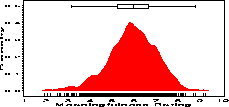boxanno Annotate a scatter plot with univariate boxplots boxanno

# SAS Macro Programs: boxanno

\$Version: 1.8 (03 Feb 2004)
Michael Friendly
York University

## BOXANNO and BOXAXIS macros (get boxanno.sas)

BOXANNO.SAS contains two SAS macros to annotate a scatterplot with marginal boxplots of one or more of the variables plotted with either PROC GPLOT, PROC G3D or (as of Version 1.5) PROC GCHART .
BOXAXIS
Creates an Annotate data set to draw a boxplot for one axis in a 2D or 3D scatterplot or a GCHART.
BOXANNO
Uses two calls to BOXAXIS to create an Annotate data set for boxplots on both axes.
Use BOXANNO to draw the boxplots for both variables in a scatterplot. For a G3D scatterplot, use one call to BOXANNO for two of the variables and BOXAXIS for the third. See the examples in "Section 4.5".

For separate use of BOXAXIS with the SAS autocall facility, you may want to make a link from boxanno.sas to boxaxis.sas in your local SASAUTOS directory. On Unix systems this would be

```  ln -s boxanno.sas boxaxis.sas
```

### Parameters for BOXAXIS

DATA=_LAST_
Name of the input data set
OUT=_DATA_
Name of the output Annotate data set
VAR=
Variable for which a boxplot is constructed
BAXIS=X
Axis on which it goes. Must be X, Y, or Z for a scatterplot, or MIDPOINT for a GCHART.
OAXIS=Y
The other axis in the plot
PAXIS=Z
The 3rd axis (ignored in GPLOT)
BOXwidth=4
Width of the box in percent of the data range
POS=98
Position of the center of the box on OAXIS in data percent. POS - BOXWIDTH/2 and POS + BOXWIDTH/2 must both be between 0 and 100.

### Example

The following example shows a density display of the distribution of rated meaningfulness for a collection of 925 English words, drawn with the density macro. The boxplot at the top of the figure is drawn using the %boxaxis macro, giving an alternative display of the distribution.
```axis1 order=(0 to .5 by .1) label=(a=90 'Density')
offset=(3,);
pattern1 v=solid c=red;
symbol1 v=none i=join c=black;

%boxaxis(data=paivio, out=boxanno, var=meaning, pos=96, boxwidth=5);
%density(data=paivio, var=meaning, out=densplot,
vaxis=axis1, haxis=axis2,
symbol=symbol1,
anno=boxanno,
plotopt=%str(areas=1));

```### Parameters for BOXANNO

DATA=_LAST_
Name of the data set to be plotted
XVAR=
Name of the horizontal variable
YVAR=
Name of the vertical variable
OUT=BOXANNO
Output Annotate data set, containing the annotations for the boxplots on both the horizontal and vertical axes.